# 2. For each function, find all critical points and use the Hessian to determine whether they...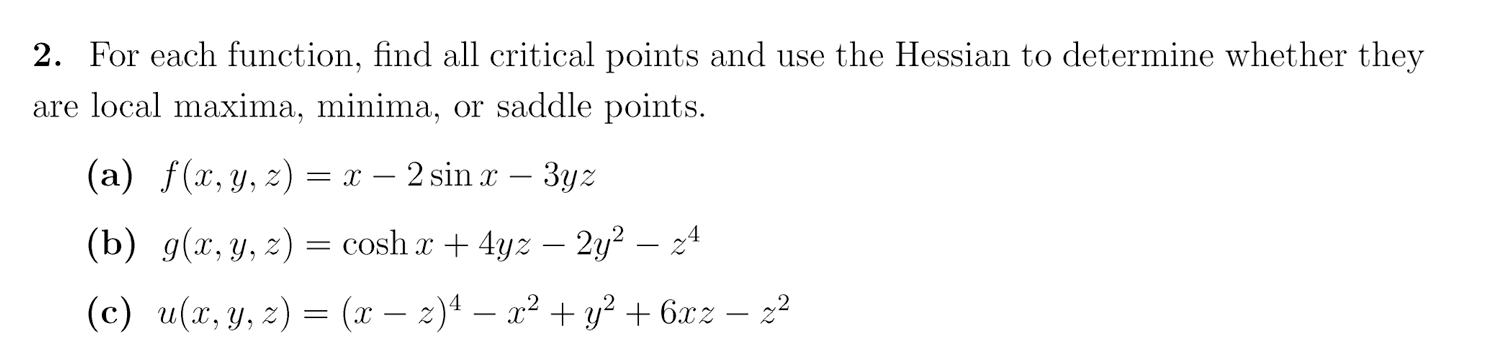2. For each function, find all critical points and use the Hessian to determine whether they are local maxima, minima, or saddle points. (a) f(x,y,z) = x — 2 sin x – 3yz (b) g(x, y, z) = cosh x + 4yz – 2y2 – 24 (c) u(x, y, z) = (x – z)4 – x2 + y2 + 6x2 – 22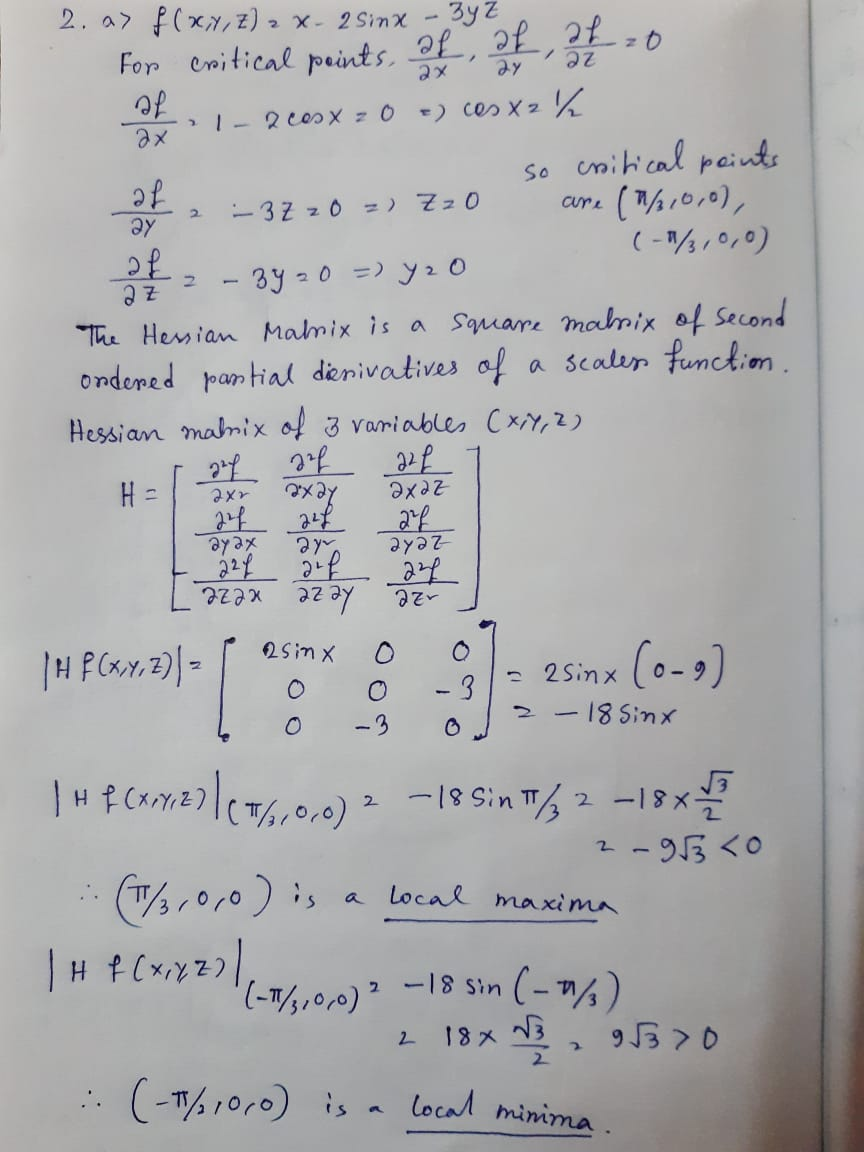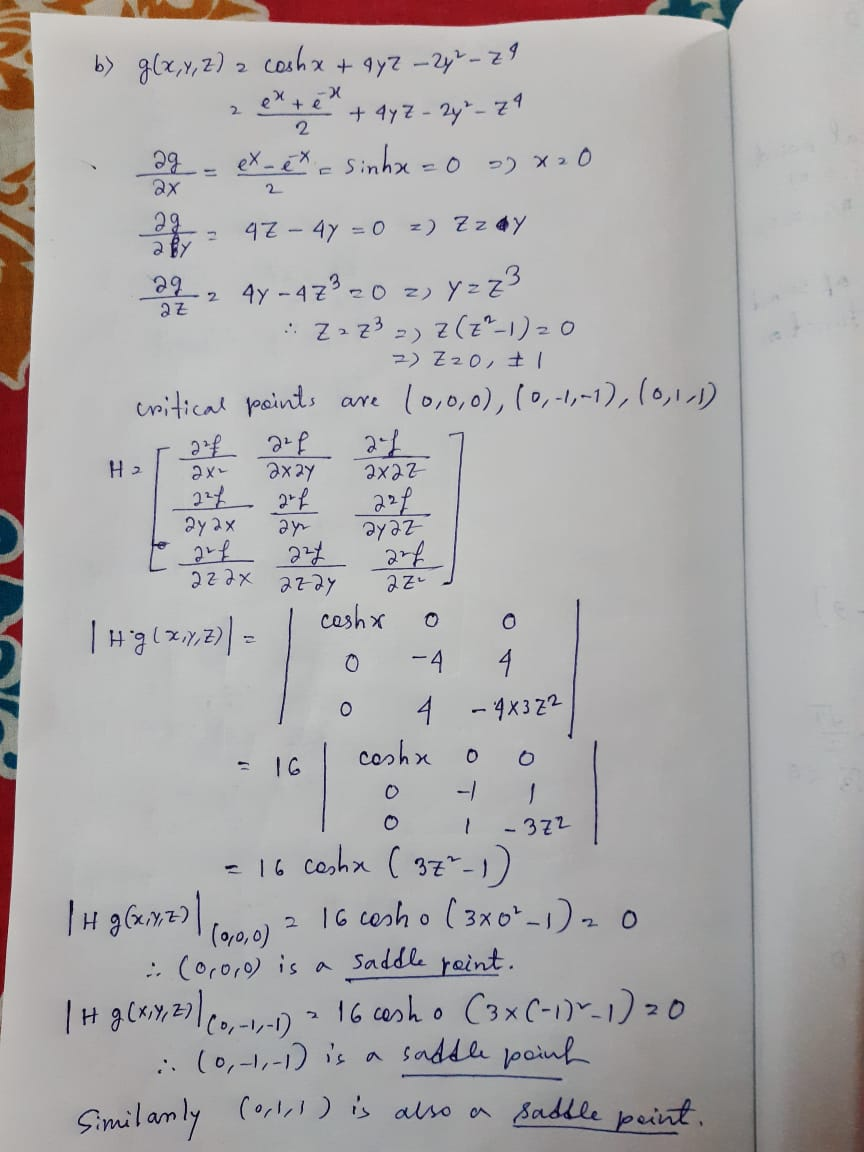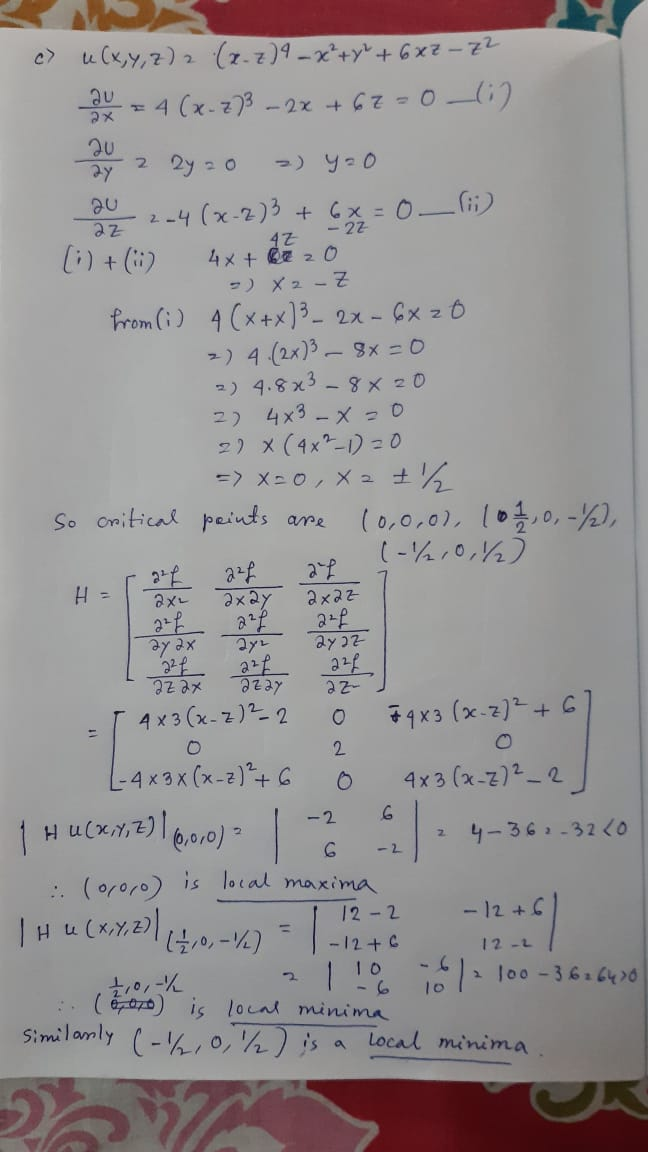##### Add Answer of: 2. For each function, find all critical points and use the Hessian to determine whether they...
Similar Homework Help Questions
• ### ECON 1111A/B Mathematical Methods in Economics II 2nd term, 2018-2019 Assignment 6 Show your step...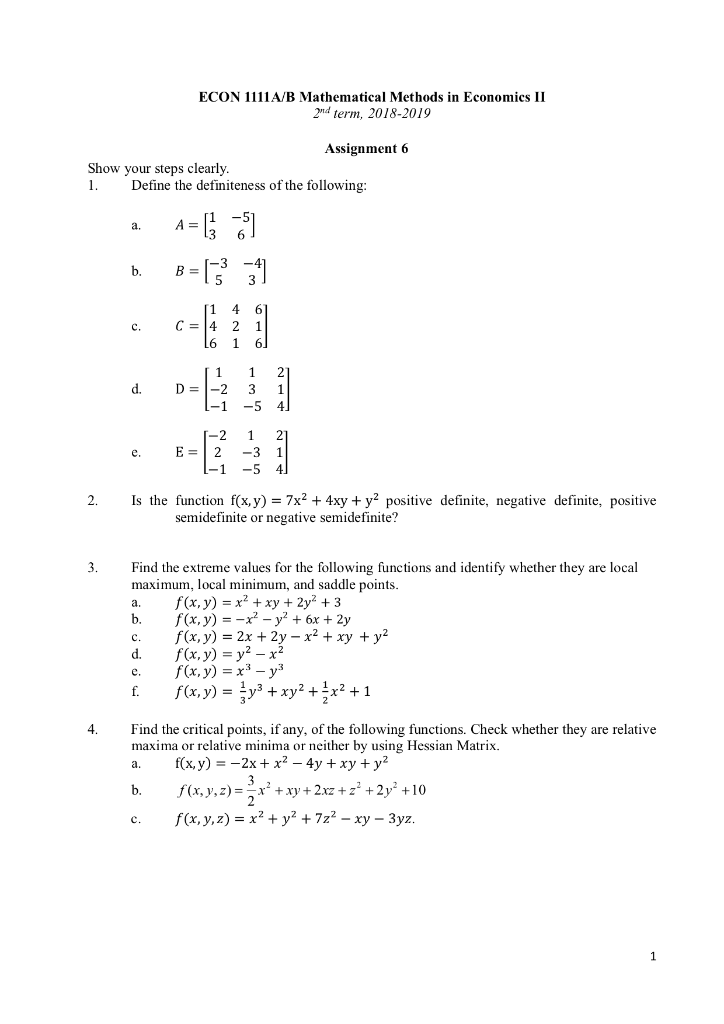ECON 1111A/B Mathematical Methods in Economics II 2nd term, 2018-2019 Assignment 6 Show your steps clearly Define the definiteness of the following A-[1 5 a. b. 1 4 6 d. D= -2 3 1 -2 1 2 E 2 -3 1 2. Is the function f(x,y) - 7x2 + 4xy + y2 positive definite, negative definite, positive semidefinite or negative semidefinite? Find the extreme values for the following functions and identify whether they are local maximum, local minimum, and saddle...

• ### Question 5 (25 points) : Use Lagrange multipliers to find all the critical points of the function f (x, y, z)-22on the surface 2 -4y2 722. You do not need to determine whether they are maxima, minima...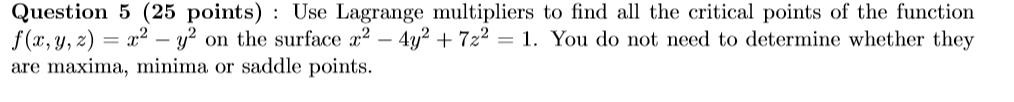Question 5 (25 points) : Use Lagrange multipliers to find all the critical points of the function f (x, y, z)-22on the surface 2 -4y2 722. You do not need to determine whether they are maxima, minima or saddle points. Question 5 (25 points) : Use Lagrange multipliers to find all the critical points of the function f (x, y, z)-22on the surface 2 -4y2 722. You do not need to determine whether they are maxima, minima or saddle points.

• ### 15. Find the critical points of the function f(x, y) = y3 - 6y? - 2x3...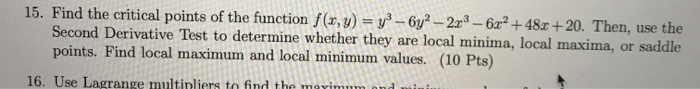15. Find the critical points of the function f(x, y) = y3 - 6y? - 2x3 - 6x2 +48x+20. Then, use the Second Derivative Test to determine whether they are local minima, local maxima, or saddle points. Find local maximum and local minimum values. (10 Pts) 16. Use Lagrange multinliers to find the maximum

• ### 4. (a) (15 points) Find all the local maxima, local minima, and saddle points of the function f(x, y)ry b) (15 points) Use Lagrange multipliers to find the points on the surface ryz-1 closest to the...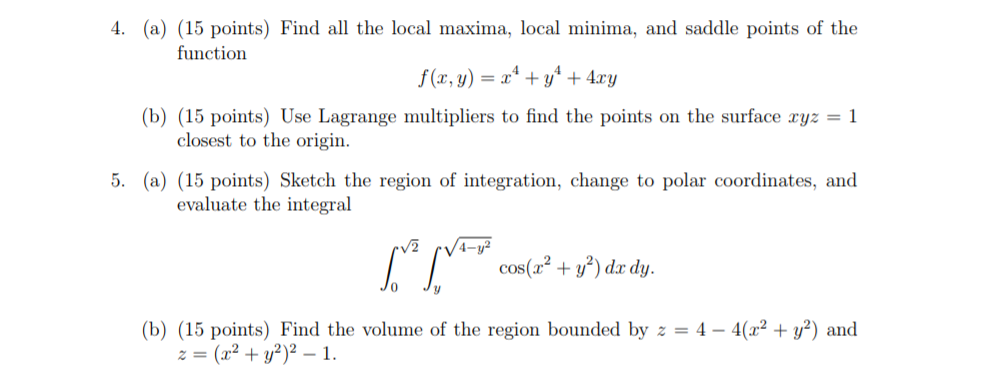4. (a) (15 points) Find all the local maxima, local minima, and saddle points of the function f(x, y)ry b) (15 points) Use Lagrange multipliers to find the points on the surface ryz-1 closest to the origin. polar coordinates, and evaluate the integral cos(a+)d dy. 0 (b) (15 points) Find the volume of the region bounded by z = 4-4(12 + y2) and z=(x2 + y2)2-1. 4. (a) (15 points) Find all the local maxima, local minima, and saddle points...

• ### Find the critical points of the following function. Use the Second Derivative Test to determine if...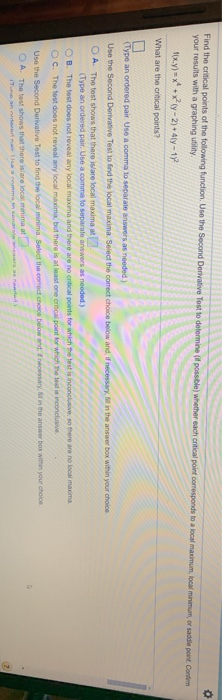Find the critical points of the following function. Use the Second Derivative Test to determine if possible) whether each critical point corresponds to a local maximum, local minimum or saddle point. Contem your results with a graphing utility f(x,y) = x + xy-2) + 4y - 12 What are the critical points? (Type an ordered pair Use a comma to separate answers as needed.) Use the Second Derivative Test to find the local maxima. Select the correct choice below and,...

• ### f(r,y)一2 + 2 ry-v2-4r-8y + 9 For Exercises 1-6, find the critical points of the given func- tion and classify them...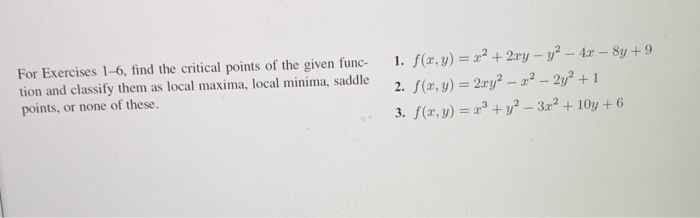f(r,y)一2 + 2 ry-v2-4r-8y + 9 For Exercises 1-6, find the critical points of the given func- tion and classify them as local maxima, local minima, saddle points, or none of these. I. 3. f(z, y) =x3 + y2-3x2 + 10y + 6 f(r,y)一2 + 2 ry-v2-4r-8y + 9 For Exercises 1-6, find the critical points of the given func- tion and classify them as local maxima, local minima, saddle points, or none of these. I. 3. f(z, y) =x3...

• ### Find and classify the critical points of these functions (that is, are they local maxima, minima,...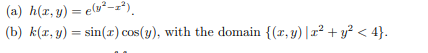Find and classify the critical points of these functions (that is, are they local maxima, minima, saddle points, or points where the function is not differentiable) (a) h(x, y) = (12-2) (b) k(x,y) = sin(I) cos(y), with the domain {(1,y) |+ y2 < 4}.

• ### Find the critical points of the following functions. Use the Second Derivative Test to determine (if...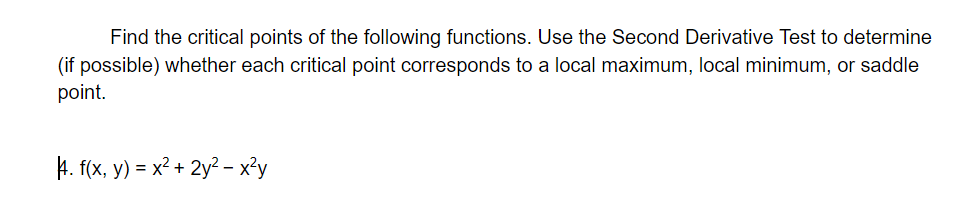Find the critical points of the following functions. Use the Second Derivative Test to determine (if possible) whether each critical point corresponds to a local maximum, local minimum, or saddle point. H. f(x, y) = x2 + 2y2 – xły

• ### (a) Find and classify all of the critical points of the function X f(x, y, z)...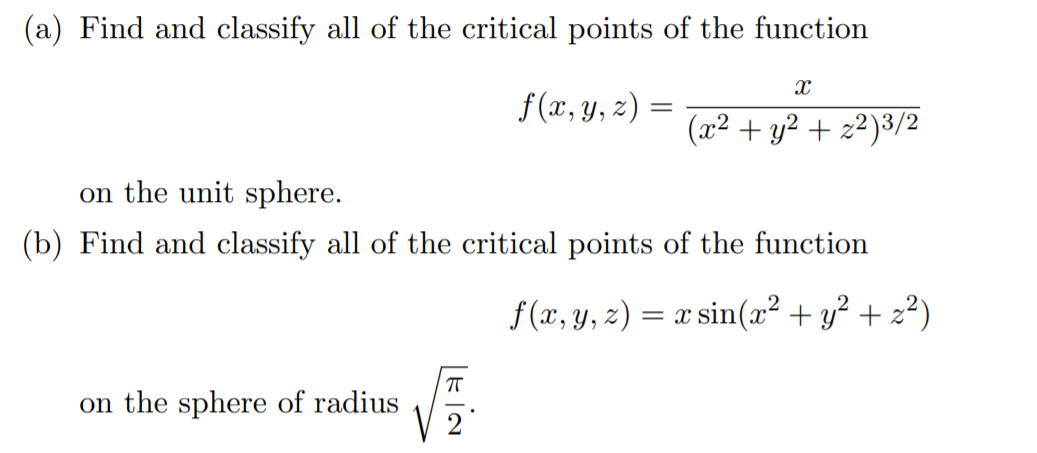(a) Find and classify all of the critical points of the function X f(x, y, z) = (x2 +42 + x2)3/2 on the unit sphere. (b) Find and classify all of the critical points of the function f(x, y, z) = x sin(x2 + y2 +22) on the sphere of radius

• ### Critical points (multivariable function)

Find the critical points and classify them as local maxima,local minima, or saddle points.f(x,y)=(x+y)(xy+1)Everytime I try to calculate this, I get something reallyweird...

Free Homework App